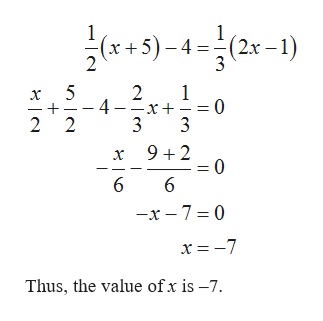How is 1/2 (x+5)-4=1/3 (2x-1) solved for an answer?

Question

How is 1/2 (x+5)-4=1/3 (2x-1) solved for an answer?

The given equation is 1/2(x...help_outlineImage Transcriptionclosex5)-4 (2x -1) 3 1 4 -x = 0 3 3 2 - 2 2 9+2 0 6 -x-7 0 x=-7 Thus, the value of x is -7. fullscreen

Want to see the full answer?

See Solution

Want to see this answer and more?

Our solutions are written by experts, many with advanced degrees, and available 24/7

See Solution
Tagged in

Algebra